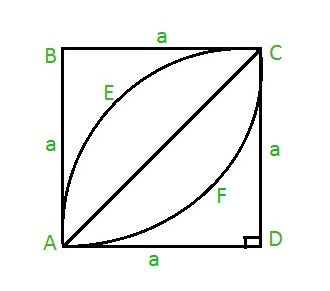Related Articles

# Area of a leaf inside a square

• Last Updated : 06 Jun, 2021

Given an integer a as the side of the square ABCD. The task is to find the area of the leaf AECFA inside the square as shown below:Examples:

Input: a = 7
Output: 28

Input: a = 21
Output: 252

Approach: To calculate the area of the leaf, first find the area of the half leaf AECA, which can be given as:

Area of half leaf = Area of quadrant AECDA – Area of right triangle ACD
Thus, Area of half leaf = ( PI * a * a / 4 ) – a * a / 2 where PI = 22 / 7 and a is the side of the square.
Hence, the area of full leaf will be ( PI * a * a / 2 ) – a * a
On taking a * a common we get, Area of leaf = a * a ( PI / 2 – 1 )

Below is the implementation of the above approach:

## C

 `// C program to find the area of``// leaf inside a square``#include ``#define PI 3.14159265` `// Function to find area of``// leaf``float` `area_leaf(``float` `a)``{``    ``return` `(a * a * (PI / 2 - 1));``}` `// Driver code``int` `main()``{``    ``float` `a = 7;``    ``printf``(``"%f"``,``           ``area_leaf(a));``    ``return` `0;``}`

## Java

 `// Java code to find the area of``// leaf inside a square``import` `java.lang.*;` `class` `GFG {` `    ``static` `double` `PI = ``3.14159265``;` `    ``// Function to find the area of``    ``// leaf``    ``public` `static` `double` `area_leaf(``double` `a)``    ``{``        ``return` `(a * a * (PI / ``2` `- ``1``));``    ``}` `    ``// Driver code``    ``public` `static` `void` `main(String[] args)``    ``{``        ``double` `a = ``7``;``        ``System.out.println(area_leaf(a));``    ``}``}`

## Python3

 `# Python3 code to find the area of leaf``# inside a square``PI ``=` `3.14159265``    ` `# Function to find the area of``# leaf``def` `area_leaf( a ):``    ``return` `( a ``*` `a ``*` `( PI ``/` `2` `-` `1` `) )``    ` `# Driver code``a ``=` `7``print``(area_leaf( a ))`

## C#

 `// C# code to find the area of``// leaf``// inside square``using` `System;` `class` `GFG {``    ``static` `double` `PI = 3.14159265;` `    ``// Function to find the area of``    ``// leaf``    ``public` `static` `double` `area_leaf(``double` `a)``    ``{``        ``return` `(a * a * (PI / 2 - 1));``    ``}` `    ``// Driver code``    ``public` `static` `void` `Main()``    ``{``        ``double` `a = 7;``        ``Console.Write(area_leaf(a));``    ``}``}`

## PHP

 ``

## Javascript

 ``
Output:
`28`

Attention reader! Don’t stop learning now. Get hold of all the important DSA concepts with the DSA Self Paced Course at a student-friendly price and become industry ready.  To complete your preparation from learning a language to DS Algo and many more,  please refer Complete Interview Preparation Course.

In case you wish to attend live classes with experts, please refer DSA Live Classes for Working Professionals and Competitive Programming Live for Students.

My Personal Notes arrow_drop_up